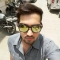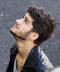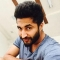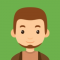## Pollard’s Rho Algorithm for Prime Factorization in javaUpdated on 25-Jun-2020 12:59:32
It is an algorithm to perform factorization on given integers. Following is the program implementing the Rho Algorithm for Prime Factorization.ProgramLive Demopublic class PollardsRho {    int num = 65;    public int gcd(int a, int b) {       int gcd = 0;       for(int i = 1; i

## Sieve of Eratosthenes in javaUpdated on 25-Jun-2020 12:55:11
Sieve of Eratosthenes is the ancient algorithm to find prime numbers up to a given number.Algorithm1. Generate integers from 2 to n (Given number).2. Counting from 2 mark every 2nd integer. (multiples of 2)3. Now, starting from 3 mark every third integer. (multiples of 3)4. Finally, marking from 5 mark every 5th integer.(multiples of 5)Programimport java.util.Scanner; public class SievePrimeFactors  {    public static void main(String args[]) {       Scanner sc = new Scanner(System.in);       System.out.println("Enter a number");       int num = sc.nextInt();       boolean[] bool = new boolean[num];       ... Read More

## Euler’s Totient function for all numbers smaller than or equal to n in javaUpdated on 25-Jun-2020 12:51:01
Following is a program to get the result of Euler’s Totient function for all numbers smaller than or equal to n when n is given.Programimport java.util.Scanner; public class EulerTotient {    public static int gcd(int a,int b){       int i, hcf = 0;          for(i = 1; i

## Euler’s criterion in javaUpdated on 25-Jun-2020 12:50:12
According to Euler’s criterion a square root of n under modulo p exists if and only if a number num exists such that num%p is equal to n%p.Programimport java.util.Scanner; public class EulersCriterion {    public static void main(String args[]) {       Scanner sc = new Scanner(System.in);       System.out.println("Enter n value :");             int n = sc.nextInt();       System.out.println("Enter p value :");       int p = sc.nextInt();       n = n % p;       int flag = 0;            for ... Read More

## Divisors of factorials of a number in javaUpdated on 25-Jun-2020 12:47:39
Following is a Java program to find the divisors of factorials of a number.Programimport java.util.Scanner; public class DivisorsOfFactorial {    public static long fact(int i) {       if(i

## Legendre’s Formula in javaUpdated on 25-Jun-2020 12:46:49
You can calculate the exponent of the largest power of a PrimeNumber that divides the factorial n! using Legendre's formula.Programimport java.util.Scanner; public class LegendresFormula {    static int Largestpower(int n, int p) {       int ans = 0;       while (n > 0) {          n /= p;          ans += n;       }       return ans;    }    public static void main (String[] args) {       Scanner sc = new Scanner(System.in);       System.out.println("Enter the n value :");     ... Read More

## Binomial Coefficient in javaUpdated on 25-Jun-2020 12:41:38
Binomial coefficient (c(n, r) or nCr) is calculated using the formula n!/r!*(n-r)!. Following is the Java program find out the binomial coefficient of given integers.Programimport java.util.Scanner; public class BinomialCoefficient {    public static long fact(int i) {       if(i

## Multiplicative order in javaUpdated on 25-Jun-2020 12:39:40
Following is a Java program which prints the multiplicative order of given numbers.import java.util.Scanner;Programpublic class MultiplicativeOrder {    public static int gcd(int num1, int num2) {       if (num2 != 0) {          return gcd(num2, num1 % num2);       } else {          return num1;       }    }    static int multiplicativeOrder(int num1, int num2) {       if (gcd(num1, num2) != 1) {          return -1;       }       int res = 1;       int p ... Read More

## Nth Catalan numbers in javaUpdated on 25-Jun-2020 12:32:38
The nth Catalan number in terms of binomial coefficients is calculated by the formula(n + k )/k where k varies from 2 to n and n ≥ 0. i.e.Cn = (2n)!/((n+1)!n!)Programpublic class NthCatalanNumber {    public static long fact(int i) {       if(i

## Catalan numbers in javaUpdated on 25-Jun-2020 12:25:10
The nth Catalan number in terms of binomial coefficients is calculated by the formula(n + k )/k where k varies from 2 to n and n ≥ 0. i.e.Cn = (2n)!/((n+1)!n!)Programpublic class CatalanNumbers {    public static long fact(int i) {       if(i